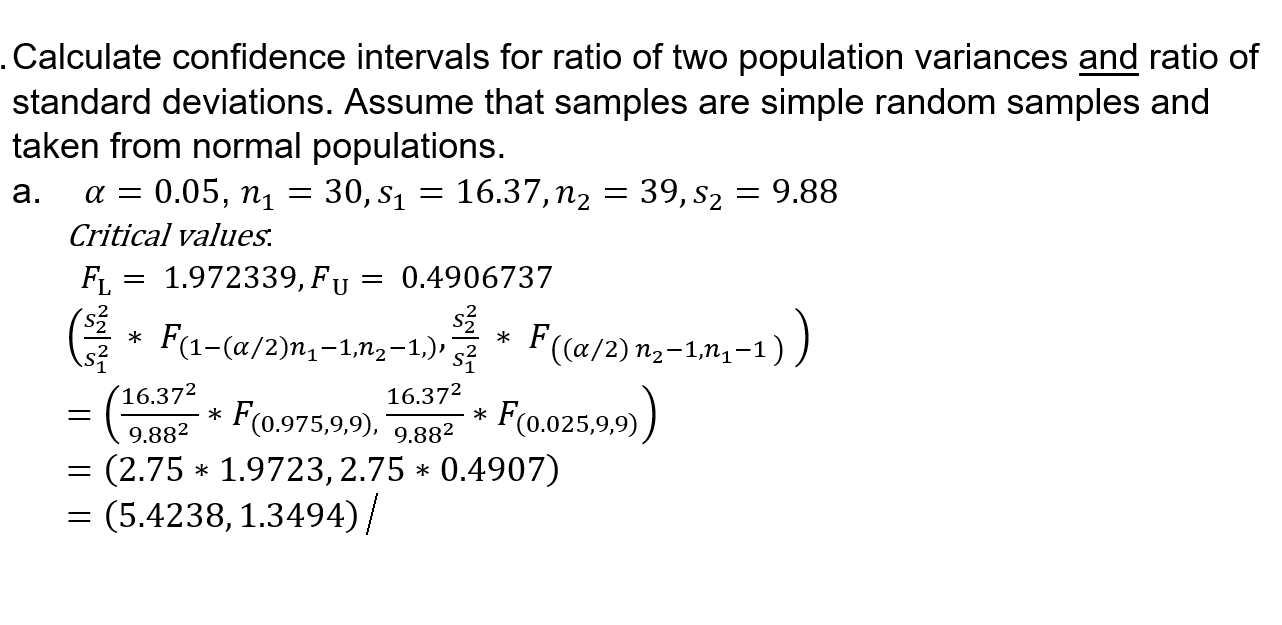# Please check my work on this problem. Do not ONLY GIVE THE CORRECT ANSWER! PLEASE SHOW...

###### Question:

Please check my work on this problem. Do not ONLY GIVE THE CORRECT ANSWER! PLEASE SHOW THE STEPS AND ANY MISTAKES I HAVE MADE! THANK YOU!!!!.Calculate confidence intervals for ratio of two population variances and ratio of standard deviations. Assume that samples are simple random samples and taken from normal populations. a. a = 0.05, n1 = 30, S1 = 16.37, n2 = 39, S2 = 9.88 Critical values. F1 = 1.972339, Fu = 0.4906737 ** F1-ſa/2n4-1.02-1.), ** F(a/2)n,-1,-1)) _ (16.372 = (49882 * F(0.975,9,9), 09.882 * F(0.025,9,9)) = (2.75 * 1.9723,2.75 * 0.4907) = (5.4238, 1.3494)/ 16.372

#### Similar Solved Questions

##### Question 2 3 pts Select the PAMPS from the list below. Select ALL that apply. RNA...
Question 2 3 pts Select the PAMPS from the list below. Select ALL that apply. RNA capsomeres capsule peptidoglycan dsDNA...
##### Which of the following items will NOT change due to the cost of financial distress? Cost...
Which of the following items will NOT change due to the cost of financial distress? Cost of legal fees Cost of goods sold Cost of equity Cost of debt...